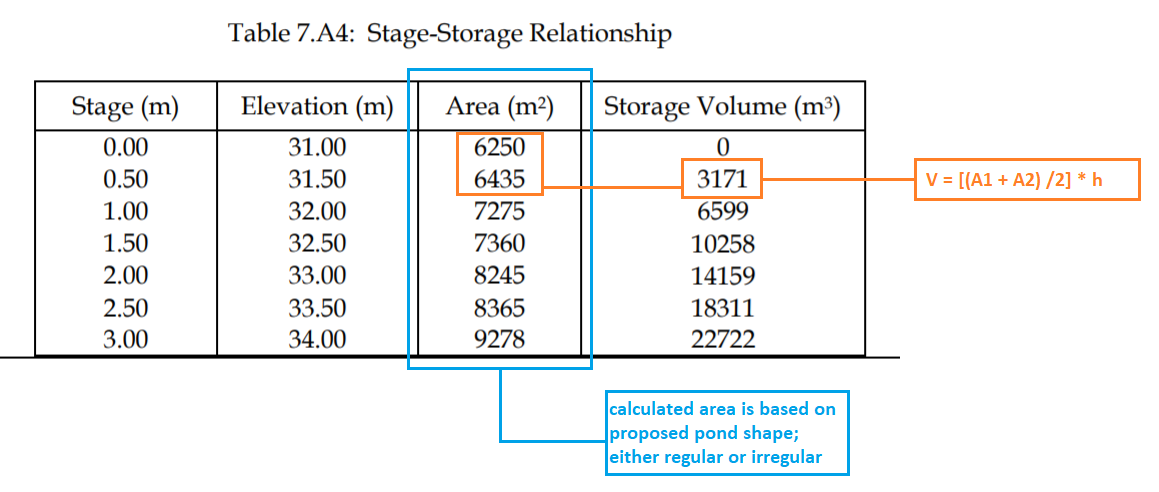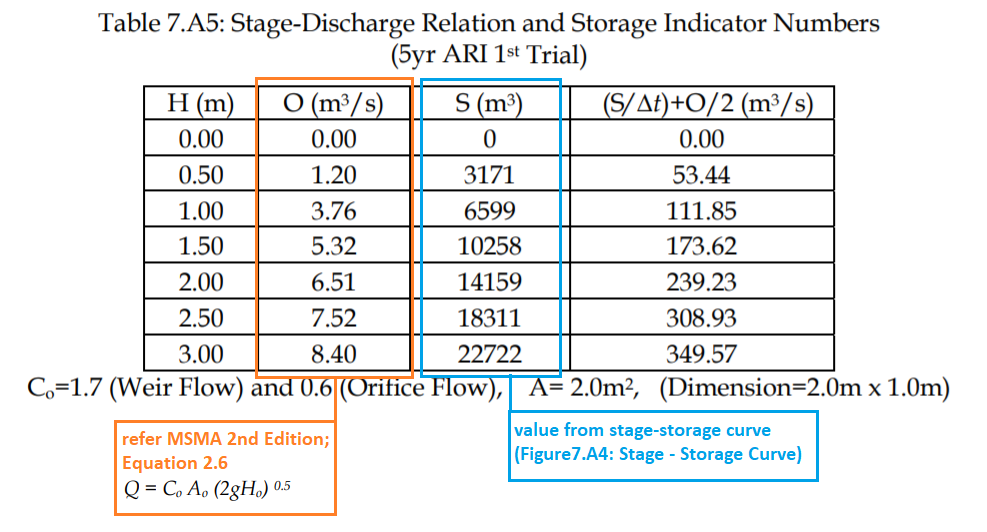603 - 5885 1250 (4 lines) info@mes100.com
Select Page

For Pond Routing, reference is made from MSMA 2nd Edition; Chapter 7 Detention Pond

MSMA 2nd Edition

### 1. Stage-Storage Relationship#### For Example; at stage 1.5m;

V = [(A1 + A2 ) / 2 ]  * 0.5

= (7275 + 7360 / 2) * 0.5

= 3658.75m3 + 6599m3

= 10257.75m3 $\approx$10258m3

### 2. Stage-Discharge Relation and Storage Indicator Numbers#### For Example: let’s take H = 1.5m,

Calculate O (m3/s) at H = 1.5m;

Note: please refer to Figure 2.8 on how to take the ‘Ho

V = Co Ao (2gHo)0.5                  (Equation 2.6 & Figure 2.8; MSMA 2nd Edition)

= 0.6 (2) ( 2 * 9.81 * (1.5-0.5))0.5

= 5.32m3/s

Calculate (S/Δt) + O/2 (m3/s)

Note: Δt is the pond routing time step, hence in this example Δt = 1min

(S/Δt) + O/2 = (10258m3 / 60sec) + 5.32m3/s

= 173.626 m3/s